## Introduction

In graphene, owing to the linear and gapless band structure, n-type (electron-like) and p-type (hole-like) regions can adjoin without a gap in between. Investigations of charge carrier transport across such a pn junction (PNJ) have revealed unique phenomena reflecting the Dirac Fermion character in graphene, such as Klein tunneling1,2,3, Veselago lensing4 and snake state5,6. In the quantum Hall (QH) effect regime under high magnetic field B, the conductance across a PNJ shows plateaus at GPNJ=G0|ν1||ν2|/(|ν1|+|ν2|), where G0=e2/h is the conductance quantum (h is Planck’s constant), ν1=2, 6, 10, … and ν2=−2, −6, −10, … are the Landau level filling factor in the n and p regions, respectively7,8,9. This conductance quantization in bipolar QH states has been explained by the mixing of counter-circulating electron and hole edge modes10,11: the current injected to the PNJ is distributed to electron and hole modes in the PNJ by the mode mixing with the ratio depending on the number of each modes, thus on ν1 and ν2, and then partitioned at the exit of the PNJ (Fig. 1b). This process gives rise to the conductance quantization at the values depending on ν1 and ν2. However, experimental study of the mode-mixing mechanism is lacking. If the mode mixing is caused by quasielastic scattering as suggested in ref. 10, 11, a graphene PNJ acts as a beam splitter of electrons and holes. A better understanding of these properties is a crucial step towards the development of electron quantum optics experiments in graphene; beam splitters together with edge states are key components for electronic interferometry12,13,14.

Shot noise measurements can provide insight into the mode-mixing mechanism (Supplementary Figs 1 and 4): when the electron and hole modes biased by Vsd are mixed, the energy distribution in the PNJ fPNJ(E) becomes out-of-equilibrium and the subsequent partitioning of the modes gives rise to the shot noise. If the mode mixing is quasielastic, fPNJ(E) is a double-step function. At zero temperature, the shot noise generated by the partitioning of the modes with double-step fPNJ(E) is expected to be (10, Supplementary Note 1),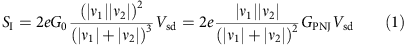characterized by the Fano factor F=SI/2eI, yielding F=0.25 for (ν1, ν2)=(2, −2). Energy losses towards external degrees of freedom can drive fPNJ(E) towards a Fermi distribution with a chemical potential eVsd/2 (Fig. 1c)15,16,17, causing the noise (and thus the Fano factor) to vanish as the carrier dwell time in the PNJ becomes larger than the energy relaxation time (18). Inelastic processes between modes in the PNJ may occur, causing fPNJ(E) to relax towards a Fermi distribution with a finite temperature Teff(Vsd) given by the balance between the Joule power dissipated in the PNJ and the heat flowing along the outgoing electronic channels10,19 (Supplementary Note 1). In this case, the Fano factor becomes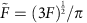[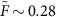for (ν1, ν2)=(2, −2)]. Note that standard transport measurements yield the same value of GPNJ for all cases, and thus cannot distinguish them.

In the following, we investigate the evolution of fPNJ(E) with carrier dwell time in the PNJ, which can be tuned by changing the length of the PNJ. We demonstrate that the amplitude of the noise is consistent with an electronic beam-splitter behaviour when PNJ is short and the energy losses are negligible.

## Results

### Measurement set-up

We obtained bipolar graphene devices using a top gate covering half of the graphene (Fig. 1a); the carrier type in the gated region can be tuned by the gate voltage VG, while that in the ungated region is fixed to electron by the doping (Methods). Therefore, the PNJ is formed at the interface between the gated and ungated regions when the carrier type in the gated region is hole for ΔVGVGVCNP<0 (VCNP is the gate voltage at the charge neutrality point). We prepared five samples with different interface lengths L=5, 10, 20, 50, and 100 μm. The direction of B is chosen so that electron and hole modes from the ohmic contacts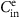and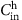, respectively, merge at the PNJ. For the noise measurement, Vsd is applied to eitherorand the noise is detected on Cdet (Fig. 1a; Supplementary Methods and Supplementary Fig. 5). Magnetic fields up to B=16 T have been applied. The base temperature is T=4.2 K.

### Detection of shot noise generated at PNJ

The inset of Fig. 2 shows the reflection of the averaged current fromto Cdet in the sample with L=50 μm. The magnetic field is B=10 T, at which the filling factor in the ungated region is fixed at νug=2. When the bipolar QH state at (νug, νg)=(2, −2) is formed for ΔVG<−10 V, the current injected fromis partitioned equally to the electron and hole modes at the exit of the PNJ, yielding a reflection of 1/2. A current noise SI appears in this regime. As Vsd applied tois increased, the excess noise ΔSISISI(Vsd=0) increases (solid cyan circles in Fig. 2). ΔSI approaches linear behaviour for eVsd>kBT, characteristic of the shot noise. A similar signal appears when Vsd is applied to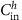(open cyan circles). In the unipolar QH state at (νug, νg)=(2, 2) for 20<ΔVG<50 V, on the other hand, the shot noise is zero (solid black circles), proving that the shot noise is indeed generated at the PNJ.

Quantitatively, we extracted F by fitting ΔSI as a function of Vsd using the relation including temperature broadening20: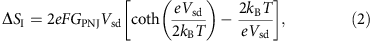where GPNJ is obtained by average current measurements. The fit yields F=0.015, which is one order of magnitude smaller than F=0.25 expected for the noise from the double-step energy distribution. This indicates that fPNJ(E) evolves during the charge propagation for L=50 μm, reducing the shot noise.

### Evolution of noise amplitude with PNJ length

The evolution of fPNJ(E) can be investigated using samples with different L. Figure 3a shows the results of the noise measurement in the bipolar QH state at (νug, νg)=(2, −2) for the five samples with L between 5 and 100 μm. The data show that the shot noise decreases with increasing L and almost disappears at L=100 μm (Fig. 3b), indicating that fPNJ(E) relaxes to the thermal equilibrium through interactions with external degrees of freedom. An exponential fit of the data yields a relaxation length L0=15 μm. The extrapolation to L=0 gives F0.27, consistent with the limit of quasielastic scattering F=0.25. Furthermore, the decrease is well reproduced by a model gradually coupling the modes propagating in the PNJ to cold external states (Supplementary Note 1 and Supplementary Fig. 2). Note that we are not able to observe whether inelastic scattering occurs inside the PNJ, because of the large error bars explained below. An important implication of the results is that, within the typical scale of usual mesoscopic devices (<1 μm), the energy loss towards external degrees of freedom is negligible and the current channels in the PNJ can be regarded as an isolated system.Figure 3: Shot noise as a function of p–n junction length.

### Fluctuation of noise

We further investigate the properties of the PNJ focusing on the energy relaxation mechanism by measuring the shot noise for a wide range of B and ΔVG. We identify the electronic states in the gated and ungated regions as a function of B and ΔVG by a low-frequency current measurement fromto Cdet (Fig. 4a) and then investigate the relation between those states and the noise. The electronic state in the ungated region depends only on B and the νug=2 QH state is formed for B>4 T. In the gated region, the non-QH states at νg=8, 4, 0 and −4 appear as a current peaks. The bipolar QH state at (νug, νg)=(2, −2) is formed for B>4 T and between νg=0 and −4 (the region indicated by dashed lines), in which the current is almost constant, consistent with the quantized conductance7,8,9. The shot noise in the sample with L=10 μm becomes small (Fig. 4b) when either or both ungated and gated regions are in a non-QH state. This confirms that shot noise is generated by the PNJ in a well-developed bipolar QH state. Within the bipolar QH state, the shot noise fluctuates largely, depending on B and ΔVG. This noise fluctuation cannot be ascribed to GPNJ, which is almost constant in the bipolar QH state. Furthermore, since the noise is generated in the well-developed QH state, the existence of multiple noise sources is unlikely. These facts indicate that the noise fluctuation is due to the fluctuation of the energy relaxation rate, which induces the fluctuation of Fano factor. Figure 4c shows the histogram of the Fano factor in the bipolar QH state calculated using equation (2). The s.d. is about 50% of the mean value.

## Discussion

The random variation of the energy relaxation rate as a function of B and ΔVG suggest that localized states in bulk graphene play a main role for the energy relaxation. Energy in the PNJ can escape to the bulk graphene through Coulomb interaction with localized states: high frequency potential fluctuations in the PNJ, which is the source of the shot noise, are dissipated in the localized states. Since the energy level and the profile of the localized states depend on B and ΔVG, fluctuations of the relaxation rate can be induced. On the other hand, the average current through the PNJ, which merely reflects the transmission coefficient, is hardly affected by the localized states. Note that a simple model of interaction with two-dimensional phonons in the PNJ fails to quantitatively reproduce our observations (Supplementary Note 1 and Supplementary Fig. 3). It is reported that the electron–phonon coupling is expected to be vanishingly small in usual unipolar edge channels21,22,23. To understand the energy relaxation length quantitatively, detailed analysis including interactions with phonons and any other possible mechanisms for the energy relaxation is necessary.

In conclusion, we showed that the mode mixing at PNJ in graphene bipolar QH states leads to non-equilibrium fPNJ(E), generating shot noise. For a short PNJ (L<<15 μm), the energy loss towards external states is negligible and the noise is consistent with a quasielastic mode mixing. This suggests that a graphene PNJ can act as a beam splitter. Since 15 μm is much larger than typical length scale of mesoscopic devices, our results encourage using graphene for electron quantum optics experiments and quantum information.

## Methods

### Device fabrication

We prepared a graphene wafer by thermal decomposition of a 6H-SiC(0001) substrate. SiC substrates were annealed at around 1,800 °C in Ar at a pressure of <100 torr. For the fabrication of devices, graphene was etched in an O2 atmosphere. After the etching, the surface was covered with 100-nm-thick hydrogen silsesquioxane (HSQ) and 60-nm-thick SiO2 insulating layers. As a result of doping from the SiC substrate and the HSQ layer, graphene has n-type carriers with the density of about 5 × 1011 cm−2. The width of the PNJ roughly corresponds to the thickness of the insulating layers and estimated to be 200 nm at most. In the QH effect regime, because of the Landau level quantization, the width becomes smaller with B. An important advantage of the SiC graphene is its size: it is single domain for 1 cm2, allowing us to investigate the effect of PNJ length.

### Noise measurement

For the noise measurement, the current noise is converted into voltage fluctuations across one 2.5 kΩ resistor in series with the sample. A 500-kHz bandwidth 3-MHz tank circuit combined with a homemade cryogenic amplifier is used. After further amplification and digitization, the autocorrelation voltage noise spectra is calculated in real-time by a computer. Accurate calibration of the noise is done using Johnson–Nyquist noise that relies on the quantification of the resistance at ν=2 and the temperature of the system.